Three-Phase Voltage Source Inverter

Three-phase voltage source inverter

• Library:
• Powertrain Blockset / Propulsion / Electric Motors and Inverters

•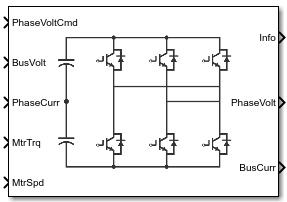Description

The Three-Phase Voltage Source Inverter block implements a three-phase voltage source inverter that generates neutral voltage commands for a balanced three-phase load. Configure the voltage switching function for continuous vector modulation or inverter switch input signals. You can incorporate the block into a closed-loop model to simulate a power inverter. The block controls the ideal switch states.

To enable power loss calculations suitable for code generation targets that limit memory, select Enable memory optimized 2D LUT. Click Calibrate Maps to virtually calibrate an inverter power loss lookup table as a function of motor torque and motor speed.

If you select , click Calibrate Maps to virtually calibrate the power loss table as a function of motor torque, motor speed, and inverter temperature. You cannot enable memory optimization for the 3D power loss lookup table.

Use the Switching voltage function parameter to set the switching voltage function.

Setting

Implementation

Illustration

Commanded phase voltage

Phase a, b, c line-to-neutral voltage command input. Suitable for continuous sinusoidal or space vector modulation input signals.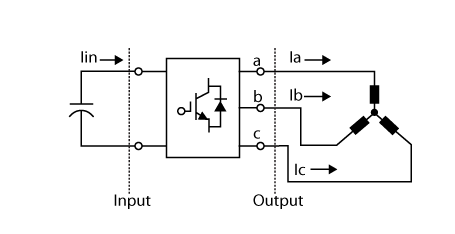Switch inputs (default)

Inverter switch input command. Suitable for hardware-in-the-loop (HIL) simulation.

The inverter switches S1, S3, and S5 using complimented control for S2, S4, and S6.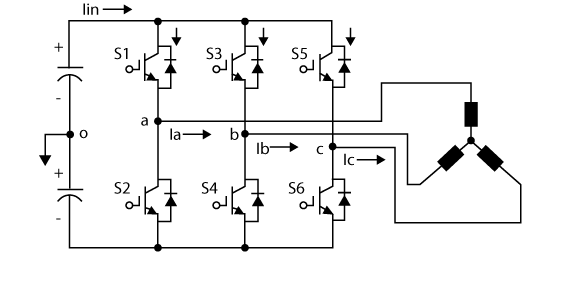Virtual Calibration

If you have Model-Based Calibration Toolbox™, click Calibrate Maps to virtually calibrate the lookup tables using measured data. The dialog box steps through these tasks.

Description

Import Loss Data

Import this loss data from a file. For example, open <matlabroot>/toolbox/autoblks/autoblksshared/mbctemplates/MappedInverterDataset.xlsx.

Input inverter temperature Setting

Required Data

off

• Motor torque, N·m

• Power loss, W

on

• Motor torque, N·m

• Motor temperature, K

• Power loss, W

Collect inverter data at steady-state operating conditions. Data should cover the inverter speed, torque, and temperature operating range.

To filter or edit the data, select . The Model-Based Calibration Toolbox Data Editor opens.

Generate Response Models

Model-Based Calibration Toolbox uses test plans to fit data to Gaussian process models (GPMs).

To assess or adjust the response model fit, select . The Model-Based Calibration Toolbox Model Browser opens. For more information, see Model Assessment (Model-Based Calibration Toolbox).

Generate Calibration

Model-Based Calibration Toolbox calibrates the response models and generates calibrated tables.

To assess or adjust the calibration, select . The Model-Based Calibration Toolbox CAGE Browser opens. For more information, see Calibration Lookup Tables (Model-Based Calibration Toolbox).

Update block parameters

Update these parameters with the calibration.

Input inverter temperature Setting

Parameters

off
• Vector of speeds (w) for tabulated losses, w_eff_bp

• Vector of torques (T) for tabulated losses, T_eff_bp

• Corresponding power loss, ploss_table

on
• Vector of speeds (w) for tabulated losses, w_eff_bp

• Vector of torques (T) for tabulated losses, T_eff_bp

• Vector of temperatures for tabulated losses, Temp_eff_bp

• Corresponding power loss, ploss_table_3d

Switching Function

For the switch voltage, the block implementation depends on the Switching voltage function setting.

SettingCalculationEquations
Commanded phase voltage

Continuous line-to-neutral voltage commands set to phase a, b, c line-to-neutral voltage command input

$\begin{array}{l}{v}_{an}={v}_{a_cmd}\\ {v}_{bn}={v}_{b_cmd}\\ {v}_{cn}={v}_{c_cmd}\end{array}$

Line-to-line voltage

Switch inputs

Switching function

Line-to-center point voltage

Line-to-neutral voltage

Line-to-line voltage

The equations use these variables.

 SFa, SFb, SFc Phase a, b, c line switching functions, respectively vbus Power source bus voltage Vao, Vbo, Vco Phase a, b, c line-to-center voltage, respectively Van, Vbn, Vcn Phase a, b, c line-to-neutral voltage, respectively Vab, Vbc, Vca Phase ab, bc, ca line-to-neutral voltage, respectively Va_cmd, Vb_cmd, Vc_cmd Phase a, b, c line-to-neutral voltage commands, respectively

Current and Power Loss

For the line-to-center, line-to-neutral, and line-to-line voltage, the block implements these equations.

CalculationEquations
Motor and bus power

Inverter power loss and bus current

The equations use these variables.

 Pmtr Power delivered to the motor Pbus Power from input bus Ploss Power loss ibus Power source bus current ia, ib, ic Phase a, b, c line current, respectively Van, Vbn, Vcn Phase a, b, c line-to-neutral voltage, respectively vbus Power source bus voltage

Power Accounting

For the power accounting, the block implements these equations.

Bus Signal DescriptionVariableEquation

PwrInfo

PwrTrnsfrd — Power transferred between blocks

• Positive signals indicate flow into block

• Negative signals indicate flow out of block

PwrMtr

Power delivered to the motor

PTrnsfrdMtr

PwrBus

Power from input bus

PTrnsfrdBus

PwrNotTrnsfrd — Power crossing the block boundary, but not transferred

• Positive signals indicate an input

• Negative signals indicate a loss

PwrLoss

Power loss

Negative value indicates power loss

PNotTrnsfrd

PwrStored — Stored energy rate of change

• Positive signals indicate an increase

• Negative signals indicate a decrease

Not used

Lookup Table Memory Optimization

The inverter power loss table parameter Corresponding power loss, ploss_table data is a function of motor torque and motor speed at different battery voltages. Positive current indicates battery discharge. Negative current indicates battery charge.

To enable power loss calculations suitable for code generation targets that limit memory, select Enable memory optimized 2D LUT. The block uses linear interpolation to optimize the inverter power loss lookup table values for code generation. This table summarizes the optimization implementation.

Use CaseImplementation

Motor speed and torque input align with the lookup table breakpoint values.

Memory-optimized power loss is power loss lookup table value at intersection of motor speed and torque.

Motor speed and torque input do not align with the lookup table breakpoint values, but are within range.

Memory-optimized power loss is linear interpolation between corresponding motor speed and torque.

Motor speed and torque input do not align with the lookup table breakpoint values, and are out of range.

Cannot compute a memory-optimized power loss. Block uses extrapolated data.

Extrapolation

The lookup tables optimized for code generation do not support extrapolation for data that is out of range. However, you can include pre-calculated extrapolation values in the power loss lookup table by selecting Specify Extrapolation.

The block uses the endpoint parameters to resize the table data.

User InputExtrapolation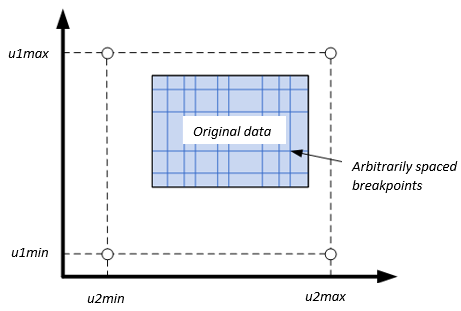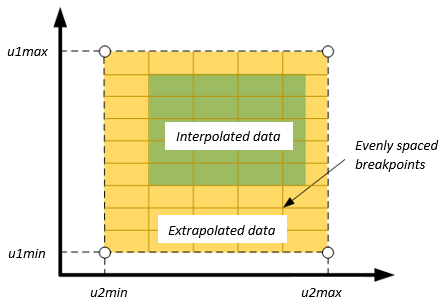Ports

Input

expand all

Phase a, b, c line-to-neutral voltage command, Va_cmd, Vb_cmd, and Vc_cmd, in V.

Dependencies

To create this port, set Switching voltage function to Commanded phase voltage.

Switch commands, Sa, Sb, and Sc, dimensionless.

Dependencies

To create this port, set Switching voltage function to Switch inputs.

Power source bus voltage, Vbus, in V.

Phase a, b, c current, ia, ib, and ic, in A.

Motor torque, Tmtr, in N·m.

Angular speed of the motor, ωmtr, in rad/s.

Inverter operating temperature, TempInvrtr, in K.

Dependencies

To create this port, select Input inverter temperature.

Output

expand all

The bus signal contains these block calculations.

Signal DescriptionVariableUnits

BusCurr

Power source bus current

ibus

A

PwrLossInv

Inverter power loss

εinv

dimensionless

PwrInfo

PwrTrnsfrd

PwrMtr

Power delivered to the motor

PTrnsfrdMtr

W

PwrBus

Power from input bus

PTrnsfrdBus

W

PwrNotTrnsfrd

PwrLoss

Power loss

PNotTrnsfrd

W

PwrStored

Not used

Phase a, b, c line-to-neutral voltage, Van, Vbn, and Vcn, in V.

Power source bus current, ibus, in A.

Parameters

expand all

Block Options

Select this parameter to create the InvrtrTemp input port.

The block enables you to specify inverter power loss lookup tables that are functions of motor torque, Tmtr, and motor speed, ωmtr. If you select , the tables are also a function of the inverter temperature, TempInvrtr.

Parameter Setting

Enables Efficiency Table

Function Of

off

Corresponding power loss, ploss_table

ƒ(Tmtr, ωmtr)

on

Corresponding power loss, ploss_table_3d

ƒ(Tmtr, ωmtr, TempInvrtr)

Dependencies

If you select to specify a 3D power loss lookup table as a function of motor torque, motor speed, and inverter temperature, you cannot select Enable memory optimized 2D LUT to enable a memory optimization.

Enable generation of memory-optimized lookup tables, suitable code generation targets that limit memory.

Dependencies

If you select Enable memory optimized 2D LUT, you cannot select .

If you have Model-Based Calibration Toolbox, click Calibrate Maps to virtually calibrate the lookup tables using measured data. The dialog box steps through these tasks.

Description

Import Loss Data

Import this loss data from a file. For example, open <matlabroot>/toolbox/autoblks/autoblksshared/mbctemplates/MappedInverterDataset.xlsx.

Input inverter temperature Setting

Required Data

off

• Motor torque, N·m

• Power loss, W

on

• Motor torque, N·m

• Motor temperature, K

• Power loss, W

Collect inverter data at steady-state operating conditions. Data should cover the inverter speed, torque, and temperature operating range.

To filter or edit the data, select . The Model-Based Calibration Toolbox Data Editor opens.

Generate Response Models

Model-Based Calibration Toolbox uses test plans to fit data to Gaussian process models (GPMs).

To assess or adjust the response model fit, select . The Model-Based Calibration Toolbox Model Browser opens. For more information, see Model Assessment (Model-Based Calibration Toolbox).

Generate Calibration

Model-Based Calibration Toolbox calibrates the response models and generates calibrated tables.

To assess or adjust the calibration, select . The Model-Based Calibration Toolbox CAGE Browser opens. For more information, see Calibration Lookup Tables (Model-Based Calibration Toolbox).

Update block parameters

Update these parameters with the calibration.

Input inverter temperature Setting

Parameters

off
• Vector of speeds (w) for tabulated losses, w_eff_bp

• Vector of torques (T) for tabulated losses, T_eff_bp

• Corresponding power loss, ploss_table

on
• Vector of speeds (w) for tabulated losses, w_eff_bp

• Vector of torques (T) for tabulated losses, T_eff_bp

• Vector of temperatures for tabulated losses, Temp_eff_bp

• Corresponding power loss, ploss_table_3d

Electrical Model

Use the Switching voltage function parameter to set the switching voltage function.

Setting

Implementation

Illustration

Commanded phase voltage

Phase a, b, c line-to-neutral voltage command input. Suitable for continuous sinusoidal or space vector modulation input signals.Switch inputs (default)

Inverter switch input command. Suitable for hardware-in-the-loop (HIL) simulation.

The inverter switches S1, S3, and S5 using complimented control for S2, S4, and S6.Vector of motor speed, ωmtr, breakpoints for power loss, in rad/s. If you set Enable memory optimized 2D LUT, the block converts the data to single precision.

Speed breakpoint storage size, n1, dimensionless. The block resamples the Corresponding power loss, ploss_table data based on the storage size.

Dependencies

To create this parameter, select Enable memory optimized 2D LUT.

Vector of motor torque, Tmtr, breakpoints for power loss, in N·m. If you set Enable memory optimized 2D LUT, the block converts the data to single precision.

Torque breakpoint storage size, n2, dimensionless. The block resamples the Corresponding power loss, ploss_table data based on the storage size.

Dependencies

To create this parameter, select Enable memory optimized 2D LUT.

Vector of inverter temperature, TempInvrtr, breakpoints for power loss, in K.

Dependencies

To create this parameter, select Input inverter temperature.

Array of values for power loss as a function of M motor speeds, ωmtr, and N motor torques, Tmtr, in W. Each value specifies the power loss for a specific combination of motor speed and motor torque. The array size must match the dimensions defined by the speed and torque vectors.

If you have Model-Based Calibration Toolbox, click Calibrate Maps to virtually calibrate the lookup table using measured data.

If you set Enable memory optimized 2D LUT, the block converts the data to single precision.

Dependencies

To create this parameter, clear Input inverter temperature.

Array of values for power loss as a function of M motor speeds, ωmtr, N motor torques, Tmtr, and L motor temperatures, TempInvrtr, in W. Each value specifies the power loss for a specific combination of motor speed, motor torque, and temperature. The array size must match the dimensions defined by the speed, torque, and temperature vectors.

If you have Model-Based Calibration Toolbox, click Calibrate Maps to virtually calibrate the lookup table using measured data.

Dependencies

To create this parameter, select Input inverter temperature.

Specify Extraction

Speed breakpoint maximum extrapolation endpoint, u1max, in rad/s.

Dependencies

To create this parameter, select Enable memory optimized 2D LUT and Specify Extrapolation.

Speed breakpoint minimum extrapolation endpoint, u1min, in rad/s.

Dependencies

To create this parameter, select Enable memory optimized 2D LUT and Specify Extrapolation.

Torque breakpoint maximum extrapolation endpoint, u2max, in rad/s.

Dependencies

To create this parameter, select Enable memory optimized 2D LUT and Specify Extrapolation.

Torque breakpoint minimum extrapolation endpoint, u2min, in rad/s.

Dependencies

To create this parameter, select Enable memory optimized 2D LUT and Specify Extrapolation.

 Lee, Byoung-Kuk and Mehrdad Ehsami. “A simplified functional simulation model for three-phase voltage-source inverter using switching function concept.” IEEE® Transactions on Industrial Electronics, Vol. 48, No. 2, pp. 309-321, April 2001.

 Ziogas, Phoivas D., Eduardo P. Wiechmann, and Victor R. Stefanovic. “A Computer-Aided Analysis and Design Approach for Static Voltage Source Inverters.” IEEE Transactions on Industrial Electronics Transactions on Industry Applications, Vol. IA-21, No. 5, September/October 1985.

Powertrain Blockset DocumentationGet trial now